## Saturday, May 20, 2006

### jaymie's growing post for this Tuesday

Question #1: Solve all 3 parts of the problem below

Jack collects baseball cards. Jack has 180 baseball cards in his collection. At the end of each month Jack buys 25 baseball cards to add to his collection.

a)Create a T-Chart showing how many baseball cards Jack has at the end of the next 4 months.

Jack will have a total of 280 cards at the end of the next 4 months.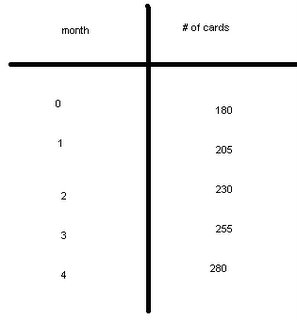b)Create an algebraic formula based upon the problem above

An algebraic formula that can be used for the problem above is:

180+25(m)=#of cards

example: 180+25(4)=280 cards

I started off with multiplying the number of months which is 4 by 25 the number of cards he buys after each month then adding his remaing 180 cards to get a total of 280 cards by then end of the next 4 months.

c)If Jack just turned 8 years old this month and Jack continues to buy the same amount of baseball cards each month, how many baseball cards will jack have when he turns 12?

Jack will have a total of 1380 cards by the the time he turns 12.

I got the answer by first figuring out the difference between 8 and 12 which is 4. So i multiplied 4 by 12 to give me 48 because there are 48 months in 4 years. Then I used the algebraic formula, 180+25(48). All I did was change the variable. So then I multiplied 48 bye 25 to get me 1200, then I added the remaining 180 to get 1380 cards.

Question #2: Use the chart to figure out the questions below.

Jackie is planning on having a pizza party with some of her friends. She is trying to figure out how many pizza’s to order. She knows that each pizza has six slices of pizza.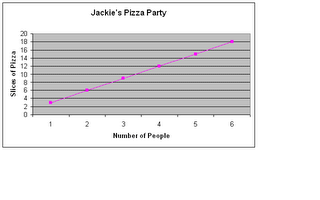a)If Jackie thinks that there will be four people at the party (counting herself) how many pizza’s should she order?

Jackie should order a total of 2 pizzas. She should order 2 pizzas because if you look at the chart and find 4 people it says that there will be 12 slices and if there are 6 slices in each pizza I divide 12 by 6 and that gives me 2 whole pizzas.

b)If two more people show up at the party how many more pizza’s does she need to order?

If two more people show up at the party Jackie should order 1 more pizza making a total of 3 pizzas all together. She should order 3 pizzas in total becuase if you look at the chart and find 6 people there will be 18 slices. So divide 18 slices by 6 to get a total of 3 pizzas.

Question #3: Answer the questions below based upon this information

Kathy has \$20 in her savings account. Each month she adds another \$15 to her savings account. In order to calculate how much money she will have in one year Kathy has created this algebraic formula: 20 + 15n = Savings amount

a) Calculate how much money Kathy will have in one year

Cathy will have \$200.00 dollars in her saving account. I got that answer by taking her formula of 20+15(12). First I mulitplied 12 by 15 to get 180 then i added her \$20.00 dollars to get \$200.00 dollars in total.

b) Suppose Kathy counted wrong and she really has \$25 in her account instead of \$20. Change the algebraic formula to reflect this miscalculation.

25+15n=savings account

Then Cathy would have a total of \$205.00 dollars for that 1 year.

Question #4: Solve the following equations. Show all of the steps that are needed.

Equations-In a equation the value of the variable is given.

a) 3n + 4n + 7 = 2n + 12

3n+4n+7=2n+12

3(1)+4(1)+7=2(1)+12

3+4+7=14

N= 1

b) 8n – (4 + 9) = 11

8n-(4+9)=11

8(3)-(4+9)=11

24-13=11

N= 3

c) (8 – 3 )n + 7n + 8 = 4n + 40

(8-3)n+7n+8=4n+40

5n+7n+8=4n+40

5(4)+7(4)+8=4(4)+40

20+28+8=56

N= 4

## Thursday, May 18, 2006

### May 18/2006 Scribe notes

For the last couple of days in math, we have been learning about patterns, graphs and algebraic formulas.

an example of what we have done is...

Jennifer's problem
Jennifer decides to open up a bank account. She starts off with \$30.00 in her account. At the end of each month she deposits \$18.00. How much will she have after 1 year?

To solve this problem we must first know how much she starts off with( \$30.00) thenn how much she puts in each month(\$18.00)and finally how long will she be putting money in her bank account( 1 year)

We start off by creating an algebraic formula: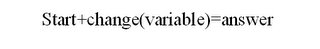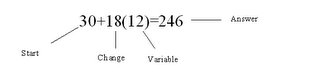to get the answer we multiply 12( 1 year) to 18(how much she is depositing) then we add the 30 dollars she has now to give us a total of \$246.00 in her bank account.

for people who do not know what a variable is it is a unknown quantity.

Then after we did the back of the worksheet Mr.Reece gave to us which said "pattern graFun" at the top.

One of the questions that was on the sheet was this question. It asks you to make an algebraic formula, draw the next shape and graph the information.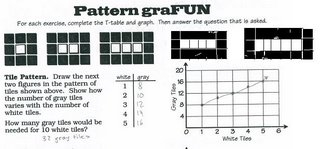The question is:

Tile Pattern. Draw the next two figures in the pattern of tiles shown above. Show how the number of gray tiles varies with the number of white tiles.

The two next figures have been already done for you. The tiles start off with a 3 by 3 box meaning that it has 9 tiles. The middle tile is white(1) then the rest are gray(8). The next box is now a 4 by 3 square which means that it now has 12 tiles. The two middle tiles are white and the remaining 10 tiles are gray. In this pattern the top and bottom rows increase by one starting off with 3. Then the white tiles increase by 1 starting from 1. Then we created a t-chart to show what found out. After, we graphed our information.

How many gray tiles would be needed for 10 white tiles.

There will be 32 gray tiles in the tenth figure. There will be 32 because in the figure that has 5 white tiles has 16 gray tiles. So if the figure with the 10 white squares is double the one with the 5 white tiles it will then has 32 gray tiles.

This worksheet containing 3 different questions will be due tomorrow, may19th/06, 4th period

After we completed that we started another worksheet that had the same kind of questions on it. That worksheet will be due at the same time as the other worksheet.

We have also started something new in class. We have started something called the growing post. The growing post is worth at least 30% of your grade. The growing post is sheets of paper with different question having to do with what we have been learing in class. The will be assigned ever monday and due the next monday. You have one week to complete it. You can either do it on blogger or Hand it in on paper with all the pictures and explanations.

Homework:

-worksheet pattern grafun
-worksheet patterns 1 problem solving -short snappers
-the growing post for the week of may 15 th to 20th due tuesday ma 23rd

No school this monday due to victoria day -jaymie-

## Wednesday, May 17, 2006

### Growing Post

Question #1: What is meant by the terms "Rotation Symmetry" and "Reflection Symmetry"? How does this relate to pentominoes? Explain these terms so that anyone who reads your post understands what you mean.

1. Rotation Symmetry- A movement of an object through turning it's figure around(1 degree-360 degrees) it's center point, giving no new shape. That is Rotation symmetry.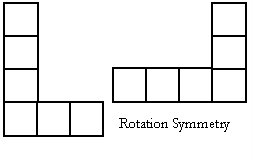Relfectfion Symmetry- The result of flipping an object. Though it may look 'unique' it is still the same as the object, it was only flipped onto the other side creating a 'reflection'.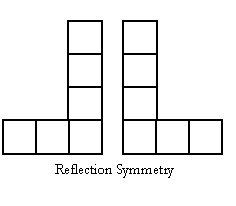Question #2: Create a pattern. You must represent this pattern both pictorially (i.e. with a picture or diagram) and with numbers. You must show the first 4 steps of your pattern.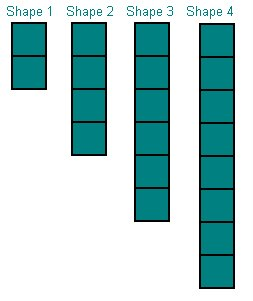Question #3: Describe in words what is occuring with your pattern and if your pattern has an ending or if it continues on indefinately. Make sure that your explanation is clear enough that the person reading your description could understand your pattern without seeing your diagram.

3. My pattern begins with two blocks, both vertical, and placed under each under. As each step grows, two block get added underneath, making the vertical line larger. This pattern would be be able to continue on forever.

Question #4: Create a T-Chart that shows what is happening with your pattern. You must show the first 4 steps of your pattern with the T-chart.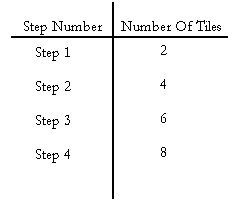[Pardon me for making this late. My internet hadn't worked on Monday. I understand that I cannot add to this post to earn the marks I could have.]

## Monday, May 15, 2006

### Janna's Growing Post

Question #1: What is meant by the terms "Rotation Symmetry" and "Reflection Symmetry"? How does this relate to pentominoes? Explain these terms so that anyone who reads your post understands what you mean.

1. "Rotation symmetry" means that if you can turn or rotate a figure or shape around the center point in less then 360 degrees and the shape doesn’t look different or changed that means that the shape has rotation symmetry.
"Reflection symmetry" means that a shape or figure will have a mirrored or flipped image of the shape that makes it look unique, but when the shape is flipped opposite they two shapes will look the same.

Rotation and reflection symmetry is related to pentominoes because there are only 12 pentominoe shapes,(a few people have found more) but if you use rotation or reflection symmetry, you will see the same pentoninoe shape you craeted but in a different position. The shape will be either rotated or flipped.

Question #2: Create a pattern. You must represent this pattern both pictorally and with numbers. You must show the first 4 steps of your pattern.

2. My pattern is below.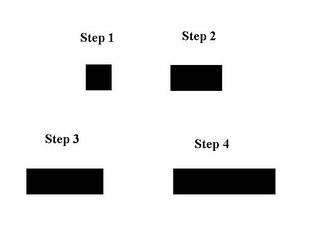Question #3: Describe in words what is occuring with your pattern and if your pattern has an ending or if it continues on indefanetly. Make sure that your explaination is clear enough that the person reading your description could understand your pattern without seeing your diagram.

In my pattern the first step starts with one block. You keep on adding a block for every step. Notice there are the same amount of blocks as there are in the step number. Example: Step number 77, there will be 77 blocks. The pattern doesn't have an end. In step 1 you have one block, in step 2 you add a block. Now you have 2 blocks. In step 3 you add another block, you now have 3 blocks In step 4 you add a block, you now have 4 blocks.

Question #4: create a T-chart that shows what is happening with your Pattern. You must show the first four steps of your pattern with the T-chart.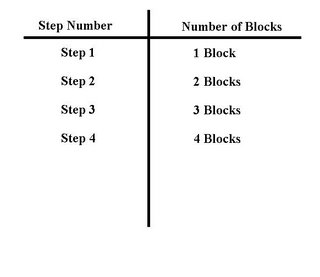### Scribe from May 8th - May 12th

Hello, fellow students. I apologize for taking a long to=ime to post the scribe but i was busy and i was making pictures. Here is the scribe below.

May 8th: We turned in corrections to our Math tests. Also, Mr. Reece gave use five(5) tiles and a sheet of grid paper per person. We were to make shapes using the five tiles. All of the shapes had to touch by one square if not more. The shapes looked like tetris shapes. Here is an example of how and how not to make the shape.
I made 12 shapes by using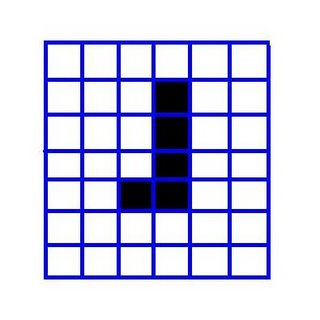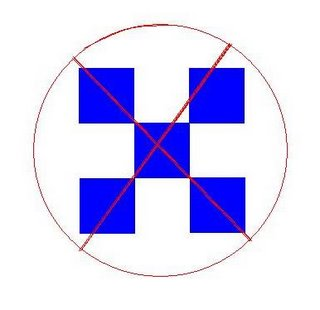I made twelve (12) different shapes. The shapes I made are below.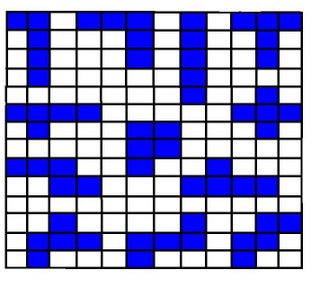May 9th: Mr.Reece gave us a sheet. the the title was "Constructing Pentominoes". The sheet is constructed below.

Constructing Pentominoes

Instructions: In your group of 2 or 3 are to construct shapes using a total of 5 tiles. Each of these five tiles must be touching at least one other tile. Once you have constructed a pentominoe you are to record the pentominoe's shape onto your piece of grid paper. You are only to record pentominoes that are unique in nature on your graph paper.

Questions for reflection

1. How many unique pentominoes were you and your partner able to create?

We answered: We were able to create 12 unique pentominoes.

2. Did you think that you would be able to discover more unique pentominoes if you had more time? How much more time would you have liked?

3. When you joined a larger group were you able to discover more unique pentominoes? How many more?

We answered: Yes, when we joined a larger group we found four more.

4. Were some of the pentominoes that you traces on your graph paper simply reflections or translations of pentominoes that you had already traced? What made these pentominoes hard to identify? Draw the perntominoes here.

We answered: yes, they were. They followed the same pattern but the outcome was different.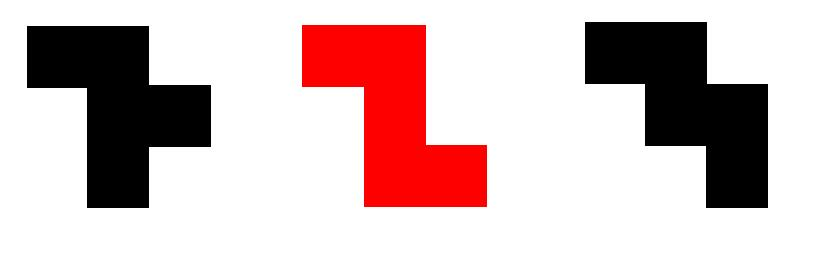5. Did you help identify pentominoes in the largergroup that initially looked different but were simply just reflections or translations of pentominoes that you already had found?

### Rustine's Growing Post

#1. What is meant by the terms "rotation symmetry" and "reflection symmetry?" How does this relate to pentominoes? Explain these terms so that anyone who reads your post understands what you mean.

rotational symmetry: is symmetry with respect to some or all rotations, has the ability to turn 360 deegres and still keep its shape.

reflection symmetry: a symmetric transformation in which a figure is reversed along an axis so that the image looks as though it is in front of a mirror.

#2 - QuestionCreate a pattern. You must represent this pattern both pictorially (i.e. with a picture or diagram) and with numbers. You must show the first 4 steps of your pattern.

The steps are listed in the picture.

#3 - QuestionDescribe in words what is occurring with your pattern and if your pattern has an ending or if it continues on indefinitely. Make sure that your explanation is clear enough that the person reading your description could understand your pattern without seeing your diagram.

In my pattern it looks like the number 'L', you begin with 5 cubes. In my pattern it adds 1 cube to the horizontal part of the letter 'L'. With every step, the horizontal part of my letter gets longer and longer. My pattern never ends .

#4 - QuestionCreate a T-Chart that shows what is happening with your pattern. You must show the first 4 steps of your pattern with the T-chart.

Mr. Reece .. i had some trouble with the pictures . SORRY .

### Kendra's Growing Post

1.Rotation symmetry is when a shape has the ability to turn 360 degrees and remain the same shape.
Reflection symmetry is when a shape has the ability to flip and remain the same shape.
Rotation and reflection symmetry relate to pentominoes because there is only a certain amount of pentominoes (12 to be exact) that are unique. if they are flipped or rotated, the pentominoes is not unique.
2.
3. You start with one square and add one square horizantaly to each step. The pattern continues.
4.

Mr. Reece it won't let me get the pictures

### Kim's Growing Post

#1. What is meant by the terms "rotation symmetry" and "reflection symmetry?" How does this relate to pentominoes? Explain these terms so that anyone who reads your post understands what you mean.

rotational symmetry: is symmetry with respect to some or all rotations. Therefore a symmetry group of rotational symmetry is a subgroup.

reflection symmetry: it is the most common type in symmetry. In 2D, there is an axis of symmetry, in 3D a plane of symmetry. An object or figure which is indistinguishable from its transformed image is called mirror symmetry.

Rotation and reflection symmetry is related to pentominoes because there are only 12 pentominoe shapes and some people have found more but if you use rotation or reflection symmetry, you will see the same pentoninoe shape but in a different position.

#3. My pattern starts with one block and you just keep adding one block more to every step and this pattern can keep on going or it can have a ending.

## Sunday, May 14, 2006

### Tony's Growing Post

Question#1
Rotational symmetry is symmetry with respect to some or all rotations in m-dimensional Euclidean space. Rotations are direct isometries, i.e., isometries preserving orientation. Therefore a symmetry group of rotational symmetry is a subgroup of E+(m) (see Euclidean group).
Symmetry with respect to all rotations about all points implies translational symmetry with respect to all translations, and the symmetry group is the whole E+(m). This does not apply for objects because it makes space homogeneous, but it may apply for physical laws.
Reflection symmetry is when you turn a shape around like a mirror

Question#2

Questions#3
For step one you have 2 blocks, for step 2 you have 3 blocks, for step 3 you have 4 and for step 4 you have 5 blocks

Question#4

Question#1

Rotation symmetry: if you rotate the shape like pentominoes it will be the same shape.

Reflection symmetry: if you flip a shape like pentominoes it will be the same shape.

Question#2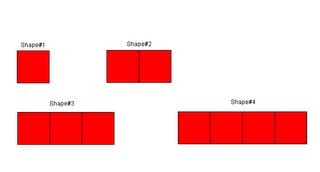Question#3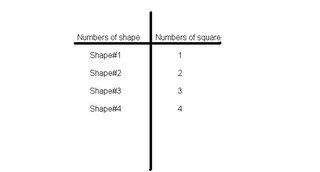<